# Weibull distribution

(diff) ← Older revision | Latest revision (diff) | Newer revision → (diff)

A particular probability distribution of random variables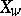, characterized by the distribution function(*)

whereis a parameter of the shape of the distribution curve,is a scale parameter andis a shift parameter. The family of distributions (*) was named after W. Weibull , who was the first to use it in the approximation of extremal data on the tensile strength of steel during fatigue testing and to propose methods for estimating the parameters of the distribution (*). Weibull's distribution belongs to the limit distributions of the third kind for the extremal terms of a series of order statistics. It is extensively used to describe the laws governing the breakdown of such things as ball bearings, vacuum instruments and electronic components. The exponential distribution (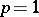) and the Rayleigh distribution () are special cases of the Weibull distribution. The distribution functions (*) do not belong to the Pearson family. There are auxiliary tables, from which the Weibull distribution functions may be calculated (). If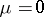, the-level quantile is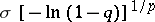,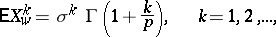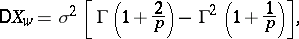where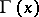is the gamma-function; the coefficients of variation, skewness and excess are independent of, which facilitates their tabulation and the compilation of auxiliary tables which can be used to obtain parameter estimates. If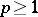, the Weibull distribution is unimodal with mode equal to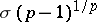, while the risk function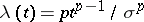is non-decreasing. If, the function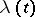monotonically decreases. It is possible to construct so-called Weibull probability paper (). On this paper,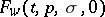becomes a straight line; if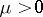, the graph of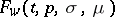is concave, and if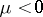it is convex. Estimates of the parameters of the Weibull distribution by the quantile method yields equations which are much simpler than those obtained by the maximum-likelihood method. The simultaneous asymptotic efficiency of the estimates of the parametersand(for) by the quantile method is maximal (and equal to 0.64) if level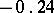and level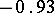quantiles are employed. The distribution function (*) is well-approximated by the distribution function of the log-normal distribution,(where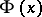is the distribution function of the standardized normal distribution and,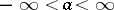,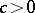):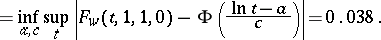How to Cite This Entry:
Weibull distribution. Encyclopedia of Mathematics. URL: http://encyclopediaofmath.org/index.php?title=Weibull_distribution&oldid=18906
This article was adapted from an original article by Yu.K. BelyaevE.V. Chepurin (originator), which appeared in Encyclopedia of Mathematics - ISBN 1402006098. See original article